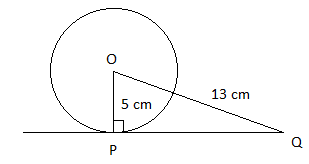QuestionAnswers

# A tangent $PQ$ at a point $P$ of a circle of radius $5$ cm meets a line through the centre $O$ at a point $Q$ so that $OQ = 13$ cm. Find the length of $PQ$.

Hint: Here the given of the problem is the radius of a circle. As any tangent and the radius of a circle is perpendicular to each other. From the given information we will get a right-angle triangle. Then we will apply the Pythagoras theorem. From the theorem, we can find the length of $PQ$.

It is given that; a tangent $PQ$ at a point $P$ of a circle of radius $5$cm meets a line through the centre $O$ at a point $Q$so that $OQ = 13$cm.We have to find the length of $PQ$.
We know that the tangent of a circle is perpendicular with its radius at the point of tangent.
So, here the radius $OP$ is perpendicular to the tangent $PQ$. So, $\angle OPQ = {90^ \circ }$.
Therefore, $\Delta OPQ$ is a right-angle triangle whose $\angle OPQ = {90^ \circ }$and $OQ = 13$cm
So, we can apply Pythagoras theorem.
We have,
$O{Q^2} = O{P^2} + P{Q^2}$
Substitute the values we get,
${13^2} = {5^2} + P{Q^2}$
Simplifying we get,
$P{Q^2} = 169 - 25$
Simplifying again we get,
$PQ = \sqrt {144} = 12$ (we will take the positive value)
Hence, the length of $PQ$ is $12$ cm.

Note: Square root of any value gives two roots: one is positive and another is negative.
Here, $PQ$ gives another value that is $- 12$. But the length of any object cannot be negative. So, we ignore negative values for the length of $PQ$.
Tangent to a circle is a line that touches the circle at one point, which is known as point of tangent. At the point of tangent, the tangent of a circle is always perpendicular to the radius.